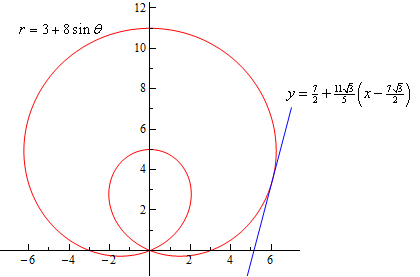Paul's Online Notes
Home / Calculus II / Parametric Equations and Polar Coordinates / Tangents with Polar Coordinates
Show Mobile Notice Show All Notes Hide All Notes
Mobile Notice
You appear to be on a device with a "narrow" screen width (i.e. you are probably on a mobile phone). Due to the nature of the mathematics on this site it is best views in landscape mode. If your device is not in landscape mode many of the equations will run off the side of your device (should be able to scroll to see them) and some of the menu items will be cut off due to the narrow screen width.

### Section 9.7 : Tangents with Polar Coordinates

We now need to discuss some calculus topics in terms of polar coordinates.

We will start with finding tangent lines to polar curves. In this case we are going to assume that the equation is in the form $$r = f\left( \theta \right)$$. With the equation in this form we can actually use the equation for the derivative $$\frac{{dy}}{{dx}}$$ we derived when we looked at tangent lines with parametric equations. To do this however requires us to come up with a set of parametric equations to represent the curve. This is actually pretty easy to do.

From our work in the previous section we have the following set of conversion equations for going from polar coordinates to Cartesian coordinates.

$x = r\cos \theta \hspace{0.75in}y = r\sin \theta$

Now, we’ll use the fact that we’re assuming that the equation is in the form $$r = f\left( \theta \right)$$. Substituting this into these equations gives the following set of parametric equations (with $$\theta$$ as the parameter) for the curve.

$x = f\left( \theta \right)\cos \theta \hspace{0.75in}y = f\left( \theta \right)\sin \theta$

Now, we will need the following derivatives.

\begin{align*}\frac{{dx}}{{d\theta }} & = f'\left( \theta \right)\cos \theta - f\left( \theta \right)\sin \theta & \hspace{0.75in} \frac{{dy}}{{d\theta }} & = f'\left( \theta \right)\sin \theta + f\left( \theta \right)\cos \theta \\ & = \frac{{dr}}{{d\theta }}\cos \theta - r\sin \theta & \hspace{0.75in} & = \frac{{dr}}{{d\theta }}\sin \theta + r\cos \theta \end{align*}

The derivative $$\frac{{dy}}{{dx}}$$ is then,

#### Derivative with Polar Coordinates

$\frac{{dy}}{{dx}} = \frac{\frac{{dr}}{{d\theta }}\sin \theta + r\cos \theta }}{\frac{{dr}}{{d\theta }}\cos \theta - r\sin \theta }$

Note that rather than trying to remember this formula it would probably be easier to remember how we derived it and just remember the formula for parametric equations.

Let’s work a quick example with this.

Example 1 Determine the equation of the tangent line to $$r = 3 + 8\sin \theta$$ at $$\displaystyle \theta = \frac{\pi }{6}$$.
Show Solution

We’ll first need the following derivative.

$\frac{{dr}}{{d\theta }} = 8\cos \theta$

The formula for the derivative $$\frac{{dy}}{{dx}}$$ becomes,

$\frac{{dy}}{{dx}} = \frac{{8\cos \theta \sin \theta + \left( {3 + 8\sin \theta } \right)\cos \theta }}{{8{{\cos }^2}\theta - \left( {3 + 8\sin \theta } \right)\sin \theta }} = \frac{{16\cos \theta \sin \theta + 3\cos \theta }}{{8{{\cos }^2}\theta - 3\sin \theta - 8{{\sin }^2}\theta }}$

The slope of the tangent line is,

$m = {\left. {\frac{{dy}}{{dx}}} \right|_{\theta = \frac{\pi }{6}}} = \frac{{4\sqrt 3 + \frac{{3\sqrt 3 }}{2}}}{{4 - \frac{3}{2}}} = \frac{{11\sqrt 3 }}{5}$

Now, at $$\theta = \frac{\pi }{6}$$ we have $$r = 7$$. We’ll need to get the corresponding $$x$$-$$y$$ coordinates so we can get the tangent line.

$x = 7\cos \left( {\frac{\pi }{6}} \right) = \frac{{7\sqrt 3 }}{2}\hspace{0.5in}y = 7\sin \left( {\frac{\pi }{6}} \right) = \frac{7}{2}$

The tangent line is then,

$y = \frac{7}{2} + \frac{{11\sqrt 3 }}{5}\left( {x - \frac{{7\sqrt 3 }}{2}} \right)$

For the sake of completeness here is a graph of the curve and the tangent line.